# 深入理解C语言指针

#### 一、指针的概念

##### 1.1、变量和地址

```void main(){
int x = 10, int y = 20;
}```

• 去酒店订了两个房间，门牌号暂时用 px、py 表示
• 让 10 住进 px，让 20 住进 py
• 其中门牌号就是 px、py 就是变量的地址
• x 和 y 在这里可以理解为具体的房间，房间 x 的门牌号（地址）是 px，房间 y 的门牌号（地址）是 py。而 10 和 20，通过 px、py 两个门牌，找到房间，住进 x、y。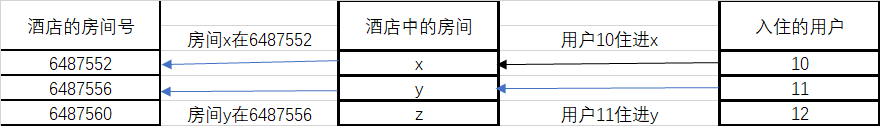#### 二、变量的指针与指针变量

##### 2.1、指针变量的定义及使用

（1）指针变量的定义

```//分别定义了 int、float、char 类型的指针变量
int *x;
float *f;
char *ch;```

（2）指针变量的使用

```//声明了一个普通变量 a
int a;
//声明一个指针变量，指向变量 a 的地址
int *pa;
//通过取地址符&，获取 a 的地址，赋值给指针变量
pa = &a;
//通过间接寻址符，获取指针指向的内容
printf("%d", *pa);```

（3）“&”和“*”的结合方向

“&”和“*”都是右结合的。假设有变量 x = 10，则*&x 的含义是，先获取变量 x 的地址，再获取地址中的内容。因为“&”和“*”互为逆运算，所以 x = *&x。

```void main(){
//声明两个普通变量
int x, y;
//声明两个指针变量
int *px, *py;
//声明一个临时变量，用于交换
int t;
//输入两个值，赋值给 x、y
scanf("%d", &x);
scanf("%d", &y);
//给指针变量 px、py 赋初值（关联变量 x、y）
px = &x;
py = &y;
//利用指针来对比 x、y 的值，如果 x 的值比 y 的值小，就交换
if(*px < *py){
//交换步骤，其中*px == x、*py == y
t = *px;
*px = *py;
*py = t;
}
printf("x =  %d, y = %d", *px, *py);
}```

```输入：23 45

##### 2.2、指针变量的初始化

```//利用取地址获取 x 的地址，在指针变量 px 定义时，赋值给 px
int x;
int *px = &x;
//定义指针变量，分别赋值"NULL"和"0"
int *p1= NULL, *p2 = 0;```
##### 2.3、指针运算

（1）赋值运算

```int *px, *py, *pz, x = 10;
//赋予某个变量的地址
px = &x;
//相互赋值
py = px;
//赋值具体的地址
pz = 4000;```

（2）指针与整数的加减运算

1. 指针变量的自增自减运算。指针加 1 或减 1 运算，表示指针向前或向后移动一个单元（不同类型的指针，单元长度不同）。这个在数组中非常常用。
2. 指针变量加上或减去一个整形数。和第一条类似，具体加几就是向前移动几个单元，减几就是向后移动几个单元。
```//定义三个变量，假设它们地址为连续的，分别为 4000、4004、4008
int x, y, z;

//定义一个指针，指向 x
int *px = &x;

//利用指针变量 px 加减整数，分别输出 x、y、z
printf("x = %d", *px);        //因为 px 指向 x，所以*px = x

//px + 1，表示，向前移动一个单元（从 4000 到 4004）
//这里要先(px + 1)，再*(px + 1)获取内容，因为单目运算符"*"优先级高于双目运算符"+"
printf("y = %d", *(px + 1));
printf("z = %d", *(px + 2));```

（3）关系运算

1. px > py 表示 px 指向的存储地址是否大于 py 指向的地址
2. px == py 表示 px 和 py 是否指向同一个存储单元
3. px == 0 和 px != 0 表示 px 是否为空指针
```//定义一个数组，数组中相邻元素地址间隔一个单元
int num = {1, 3};

//将数组中第一个元素地址和第二个元素的地址赋值给 px、py
int *px = &num, *py = &num;
int *pz = &num;
int *pn;

//则 py > px
if(py > px){
printf("py 指向的存储地址大于 px 所指向的存储地址");
}

//pz 和 px 都指向 num
if(pz == px){
printf("px 和 pz 指向同一个地址");
}

//pn 没有初始化
if(pn == NULL || pn == 0){
printf("pn 是一个空指针");
}```

#### 三、指针与数组

##### 3.1、指向数组的指针

`int nums, *p;`

```//数组名即为数组的首地址
p = nums;
//数组第一个元素的地址也是数组的首地址
p = &nums;```

*p = 1，此操作为赋值操作，即将指针指向的存储空间赋值为 1。此时 p 指向数组 nums 的第一个元素，则此操作将 nums 第一个元素赋值为 0，即 nums = 1。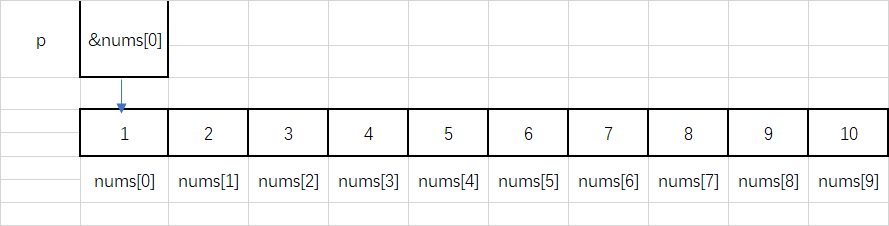p + 1，此操作为指针加整数操作，即向前移动一个单元。此时 p + 1 指向 nums的下一个元素，即 nums。通过p + 整数可以移动到想要操作的元素（此整数可以为负数）。

```//定义一个整形数组，并初始化
int nums = {4, 5, 3, 2, 7};

//定义一个指针变量 p，将数组 nums 的首地址赋值给 p，也可以用p = &nums赋值
int *p = nums, i;            //i 作为循环变量

//p 指向数组第一个元素（数组首地址），我们可以直接用间接寻址符，获取第一个元素的内容
printf("nums = %d\n", *p);            //输出结果为 nums = 4

//我们可以通过"p + 整数"来移动指针，要先移动地址，所以 p + 1 要扩起来
printf("nums = %d\n", *(p + 1));        //输出结果为 nums = 5

//由上面推导出*(p + i) = nums[i]，所以我们可以通过 for 循环变量元素
for(i = 0; i < 5; i++){
printf("nums[%d] = %d", i, *(p + i));
}```

##### 3.2、字符指针与字符数组

（1）字符数组方式

```char word[] = "zack";
printf("%s", word);```

（2）字符指针方式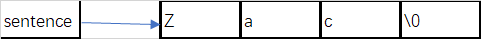```//除了定义一个字符数组外，还可以直接定义一个字符指针存储字符串
char *sentence = "Do not go gentle into that good night!";

//此时可以做字符串的操作
//输出
printf("%s", sentence);

//通过下标取字符
printf("%c", sentence);

//获取字符串长度，其中 strlen 是 string.h 库中的方法
printf("%d", strlen(sentence));```

```//定义字符数组 sentence 和 word，给 sentence 赋初值
char sentence[] = "Do not go gentle into that good night!", word;

//定义字符指针，指向 word
char *ch = word;
int i;

//循环赋值
for(i = 0; sentence[i] != '\0'; i++){
*(ch + i) = sentence[i];
}

//在当 i 等于 sentence 的长度（sentence 的长度不包含'\0'）时，
//i 继续自增，此时判断 sentence != '\0'不符合，跳出循环，则 i 比 sentence 长度大 1
*(ch + i) = '\0';

//输出字符串，因为 ch 指向 word，所以输出结果是一样的
printf("ch = %s, word = %s", ch, word);```

3.3、多级指针及指针数组

（1）多级指针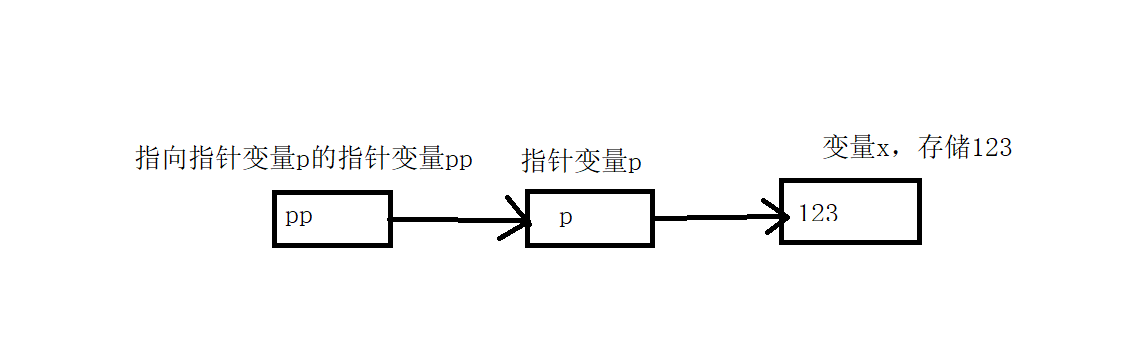`数据类型 **二级指针名;`

```//定义普通变量和指针变量
int *pi, i = 10;
//定义二级指针变量
int **ppi;

//给指针变量赋初值
pi = &i;

//给二级指针变量赋初值
ppi = &pi;

//我们可以直接用二级指针做普通指针的操作
//获取 i 的内容
printf("i = %d", **ppi);
//获取 i 的地址
printf("i 的地址为%d", *ppi);```

（2）指针数组

`数据类型 *数组名[指针数组长度];`

```//定义一个数组
int nums = {2, 3, 4, 5, 2}, i;

//定义一个指针数组
int *p;

//定义一个二级指针
int **pp;

//循环给指针数组赋值
for(i = 0; i < 5; i++){
p[i] = &nums[i];
}

//将指针数组的首地址赋值给 pp，数组 p 的数组名作为 p 的首地址，也作为 p 中第一个元素的地址。
//数组存放的内容为普通变量，则数组名为变量的指针；数组存放的内容为指针，则数组名为指针的指针。
pp = p;

//利用二级指针 pp 输出数组元素
for(i = 0; i < 5; i++){
//pp == &p == &&nums，nums == *p == **pp
printf("%d", **pp);

//指针变量+整数的操作，即移动指针至下一个单元
pp++;
}```

#### 3.4、指针与多维数组

##### （1）多维数组的地址

```int nums = {
{1, 2},
{2, 3}
};```

1. 先是第一个维度，将数组当成一种数据类型 x，那么二维数组就可以当成一个元素为 x 的一维数组。
2. 如上面的例子，将数组看成数据类型 x，那么 nums 就有两个元素。nums和 nums。
3. 我们取 nums分析。将 nums看做一个整体，作为一个名称可以用 x1 替换。则 x1就是 nums，其值为 1。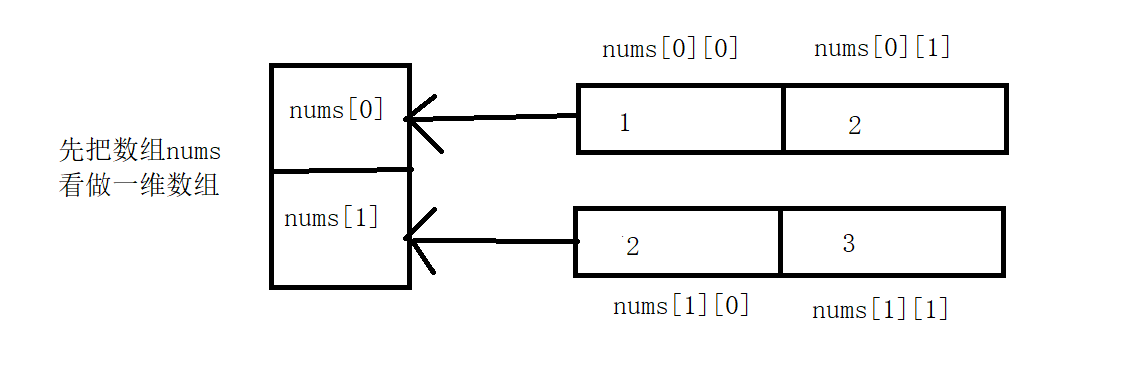`printf("%d", nums);`

```//假设已初始化，二维数组数据类型设为 x，一维数组数据类型设为 y
int nums;

//此数组首地址为该数组名称
printf("此数组首地址为%d", nums);

//此数组可以看做存储了两个 x 类型元素的一维数组，则 nums = x1 的地址为
printf("第二个维度的首地址为%d", nums);

//而 x1 可以看做存储了两个 y 类型元素的一维数组，则 y1 = x1 = nums
printf("第三个维度的首地址为%d", nums);```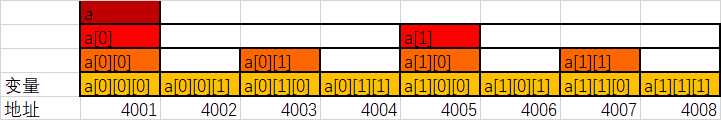`a == a == a == &a;`

##### （2）多维数组的指针

```int nums = {2, 4, 5, 6, 7};
int *p = nums;```

```//定义一个二维数组
int nums = {
{1, 2},
{2, 3}
};

//此时 nums、和 nums各为一个数组
int *p = {nums, nums};

//我们可以用指针数组 p 操作一个二维数组

//p 为数组 p 的首地址，p = nums = *p，**p = nums
printf("nums = %d", **p);

//指针 + 整数形式，p+1 移动到 nums 的地址，*(p +1) = nums，则**(p + 1) = nums
printf("nums = %d", **(p + 1));

//先*p = nums，再*p + 1 = &nums，最后获取内容*(*p + 1)即为 nums
printf("nums = %d", *(*p + 1));```

#### 四、指针与函数

##### 4.1、函数参数为指针

```void swap(int *x, int *y);
void main(){
int x = 20, y = 10;
swap(&x, &y);
printf("x = %d, y = %d", x ,y);
}
void swap(int *x, int *y){
int t;
t = *x;
*x = *y;
*y = t;
}```

##### 4.2、函数的返回值为指针

```数据类型 *函数名(参数列表){
函数体
}
//例如：
int s;
int *sum(int x, int y){
s = x + y;
return &s;
}```

```int s;
void mian(){
int *r = sum(10, 9);
printf("10 + 9 + %d", *r);
}
int *sum(int x, int y){
s = x + y;
return &s;
}```

##### 4.3、指向函数的指针

C 语言中，函数不能嵌套定义，也不能将函数作为参数传递。但是函数有个特性，即函数名为该函数的入口地址。我们可以定义一个指针指向该地址，将指针作为参数传递。

`数据类型 (*函数指针名)();`

```#include <string.h>
/**
* 定义一个方法，传入两个字符串和一个函数指针 p，用 p 对两个字符串进行操作
*/
void check(char *x, char *y, int (*p)());
void main(){
//string.h 库中的函数，使用之前需要声明该函数。字符串比较函数
int strcmp();
char x[] = "Zack";
char y[] = "Rudy";

//定义一个函数指针
int (*p)() = strcmp;

check(x, y, p);
}
void check(char *x, char *y, int (*p)()){
if(!(*p)(x, y)){
printf("相等");
}else{
printf("不相等");
}
}```

`(*p)(x, y);`